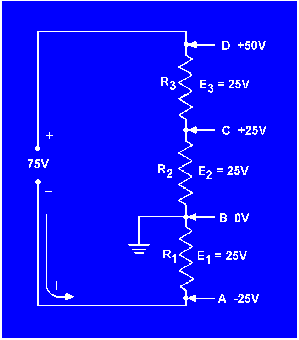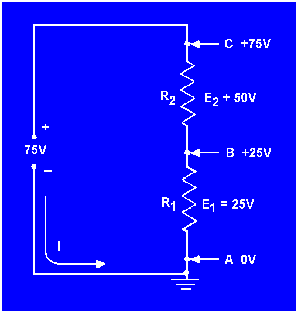Circuit terms and characteristicsCustom SearchSeries Aiding and Opposing SourcesMatter, Energy, and ElectricityOpen circuitCIRCUIT TERMS AND CHARACTERISTICS

Before you learn about the types of circuits other than the series circuit, you should become familiar with some of the terms and characteristics used in electrical circuits. These terms and characteristics will be used throughout your study of electricity and electronics.

REFERENCE POINT
A reference point is an arbitrarily chosen point to which all other points in the circuit are compared. In series circuits, any point can be chosen as a reference and the electrical potential at all other points can be determined in reference to that point. In figure 3-28 point A shall be considered the reference point. Each series resistor in the illustrated circuit is of equal value. The applied voltage is equally distributed across each resistor. The potential at point B is 25 volts more positive than at point A. Points C and D are 50 volts and 75 volts more positive than point A respectively.

Figure 3-28. - Reference points in a series circuit.When point B is used as the reference, as in figure 3-29, point D would be positive 50 volts in respect to the new reference point. The former reference point, A, is 25 volts negative in respect to point B.

Figure 3-29. - Determining potentials with respect to a reference point.As in the previous circuit illustration, the reference point of a circuit is always considered to be at zero potential. Since the earth (ground) is said to be at a zero potential, the term GROUND is used to denote a common electrical point of zero potential. In figure 3-30, point A is the zero reference, or ground, and the symbol for ground is shown connected to point A. Point C is 75 volts positive in respect to ground.

Figure 3-30. - Use of ground symbols.In most electrical equipment, the metal chassis is the common ground for the many electrical circuits. When each electrical circuit is completed, common points of a circuit at zero potential are connected directly to the metal chassis, thereby eliminating a large amount of connecting wire. The electrons pass through the metal chassis (a conductor) to reach other points of the circuit. An example of a chassis grounded circuit is illustrated in figure 3-31.

Figure 3-31. - Ground used as a conductor.Most voltage measurements used to check proper circuit operation in electrical equipment are taken in respect to ground. One meter lead is attached to a grounded point and the other meter lead is moved to various test points. Circuit measurement is explained in more detail in NEETS Module 3.Integrated Publishing, Inc.